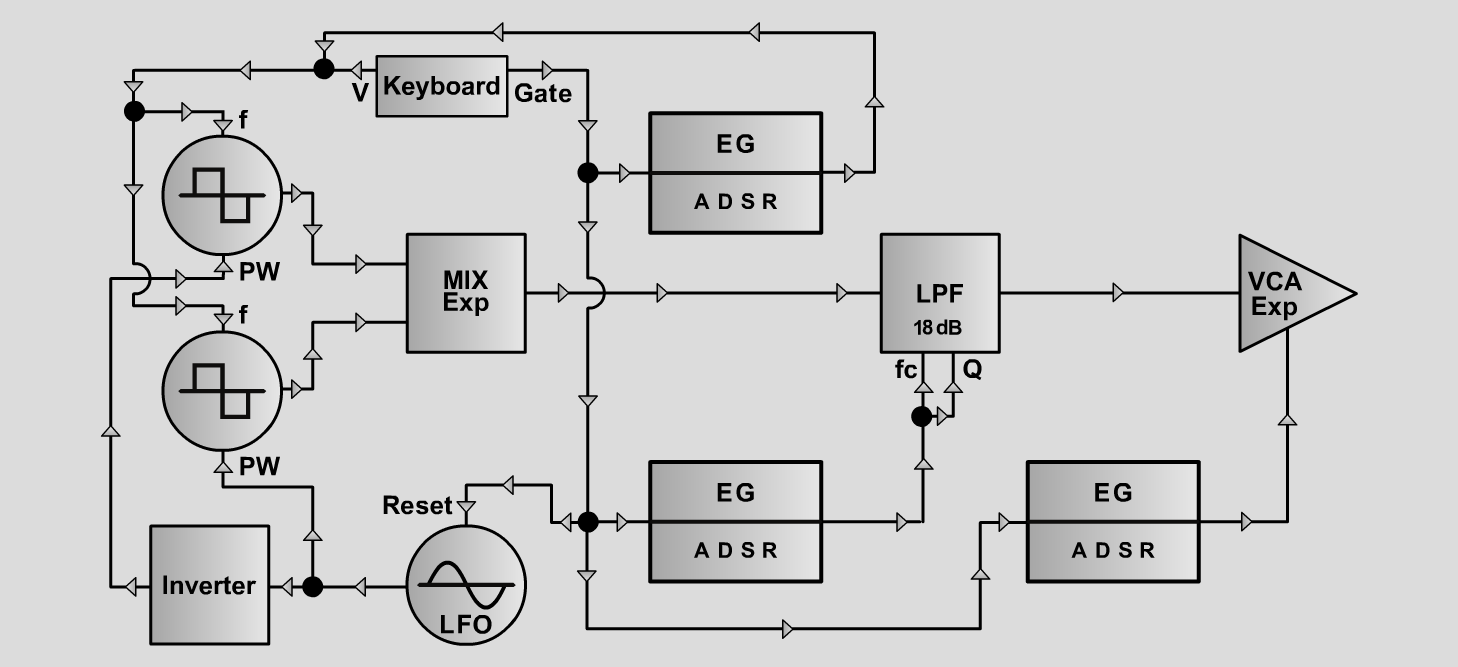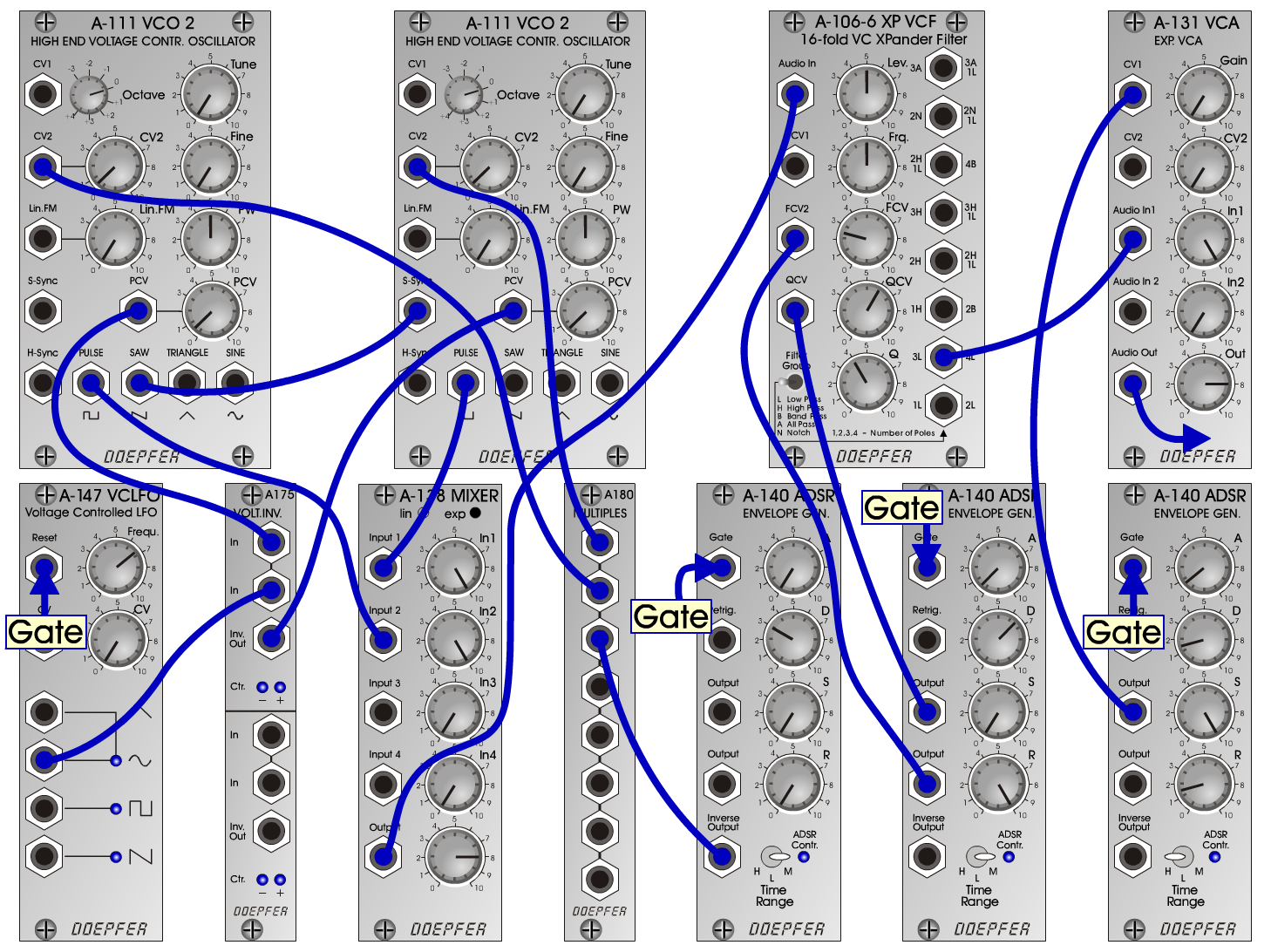S u n - S i g hS y n t h- P a dD o e p f e r A - 100 Connections: Settings Sun-Sigh A: A-111/1 (Pulse) <=> A-138 (Input 1)  A-111/2 (Pulse) <=> A-138 (Input 2)  A-111 (Saw) <=> A-111 (S-Sync)  A-138 (Output) <=> A-106-6 (Audio In)  A-106-6 (3L) <=> A-131 (Audio In)  A-140/1 (Output) <=> A-131 (CV1)  A-140/2 (Output) <=> A-106-6 (FCV2)  A-140/2 (Output) <=> A-106-6 (QCV)  A-140/3 (Inverse Output) <=> A-180  A-180 <=> A-111/1 (CV2)  A-180 <=> A-111/2 (CV2)  A-147 (Sine) <=> A-175 (In)  A-175 (In) <=> A-111/1 (PCV)  A-175 (Inv Out) <=> A-111/2 (PCV)  Gate <=> A-147 (Reset) A-111/1 (Octave = 0, CV 2 = 0.5, PW = 5, PCV = 0.5)  A-111/2 (Octave = 0, CV 2 = 0.5, PW = 5, PCV = 0.5)  A-106-6 (Lev = 5, Frq = 5, FCV = 2.5, QCV = 6, Q = 4)  A-147 (Freq = 6.7)  A-140/1 (A = 0.7, D = 1.5, S = 10, R = 1.5, Range = H)  A-140/2 (A = 0.5, D = 6.5, S = 0, R = 10, Range = M)  A-140/3 (A = 0, D = 3, S = 0, R = 0, Range = M)  A-138 (In 1 = 10, In 2 = 10, Out = 8)  A-131 (Gain = 0, Audio In 1 = 10, Audio Out = 8) Sun-Sigh B: A-111/1 (Octave = -1)  A-111/2 (Octave = 0 Sun-Sigh C: A-111/1 (Octave = -1)  A-111/2 (Octave = +1 Sun-Sigh D: A-111/1 (Octave = 0)  A-111/2 (Octave = 0  A-106-6 (Frq = 6) Sun-Sigh E: A-111/1 (Octave = -1)  A-111/2 (Octave = 0  A-106-6 (Frq = 6) Sun-Sigh F: A-111/1 (Octave = -1)  A-111/2 (Octave = +1)  A-106-6 (Frq = 6)Sound samples Bach: Gute Nacht     (from 'Jesu, meine Freude', BWV 227) Sun-Sigh A 1 Sun-Sigh A 2 Sun-Sigh A 3 Sun-Sigh B 1 Sun-Sigh B 2 Sun-Sigh B 3 Sun-Sigh C 1 Sun-Sigh C 2 Sun-Sigh C 3 Sun-Sigh D 1 Sun-Sigh D 2 Sun-Sigh D 3 Sun-Sigh E 1 Sun-Sigh E 2 Sun-Sigh E 3 Sun-Sigh F 1 Sun-Sigh F 2 Sun-Sigh F 3